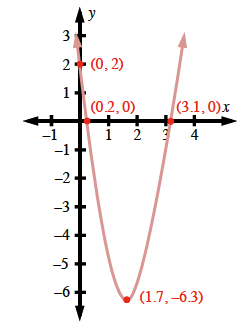### Home > CCAA8 > Chapter 11 Unit 12 > Lesson CCA: 11.3.4 > Problem11-120

11-120.

Graph the parabola described by the following equation by finding its $x$-intercepts and vertex.

$y=3x^2-10x+2$

First, convert the equation to graphing form.

$\frac{y}{3}=x^2-\frac{10x}{3}+\frac{2}{3}$

$\frac{y}{3}+\frac{19}{9}=x^2-\frac{10}{3}+\frac{25}{9}$

$y=3\left(x-\frac{5}{3}\right)^2-\frac{19}{3}$

The equation helps you find the coordinates of the vertex.

$\left(\frac{5}{3},-\frac{19}{3}\right)$

Substitute $0$ for $y$ to find the $x$-intercepts.
Substitute $0$ for $x$ to find the $y$-intercept.

$\left(\frac{5+\sqrt{19}}{3}\approx3.1,0\right)\left(\frac{5-\sqrt{19}}{3}\approx0.2,0\right)\left(0,2\right)$